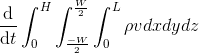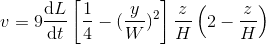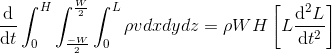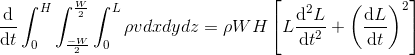# Evaluating triple integral

Hi,
I'm having a problem in evaluating a triple integral for a deformable control volume equation:where v is defined as:When I evaluate the triple integral in Maple and by hand I get:Can someone please explain where the last term on the RHS comes from??

David

arildno
$$\frac{d}{dt}(\rho{WH}L\frac{dL}{dt})$$